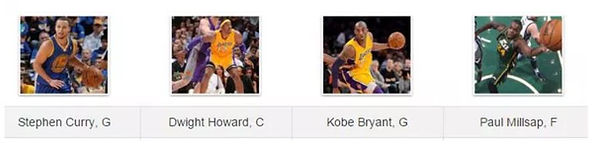## Day 64This activity came from Mathalicious.  Mathalicious is site loaded with engaging math activities.  You must pay to get access to the files, but Mathalicious allows a “pay what you can” for teachers who have to pay out of pocket.  In this activity, students will be trying to decide whether it is best to shoot 2-pointers, 3-pointers, or free throws for four different NBA players.  They investigate the question by calculating some probabilities and then using these probabilities to find expected values (of discrete random variables).  We found that we could show the students how to do each calculation for Stephen Curry and then they worked together to do the calculations for the other three players.  By the way, Stephen Curry’s 3-pointer efficiency is unparalleled in the history of the NBA.

##### Teaching Tip:

In the activity, students have to calculate the probability that Stephen Curry makes one of two free throws.  This can be found by finding the probability that he makes, then misses (0.9 X 0.1 = 0.09) and adding the probability that he misses, then makes (0.1 X 0.9 = 0.09).  So why do we multiply for the first part of the calculation and then add for the last part of the calculation?  We multiply because we want to know the probability of him making the first AND missing the second.  We add because he could make one free throw by making then missing OR missing then making.  Read: multiply probabilities for AND problems (assuming independence) and add probabilities for OR problems (assuming mutually exclusive).

This idea of making one free throw out of two will also set the stage for tomorrow’s activity, which ultimately leads us to the Binomial Formula in Lesson 5.3.